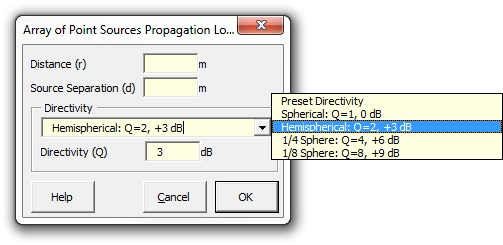### Strutt Help

Array of Point Sources Propagation Loss    1/1

Strutt|Propagation|Array of Point Sources provides the correction for sound radiation from an array of point sources, given the perpendicular distance from the source (r) in metres.Distance correction can be calculated for an infinite array of incoherent point sources by:

L_p = L_W - 6 - 10log_10 r - 10log_10 b

Where b is the distance between the separate point sources.
Note L_W is the sound power level (dB) of the individual point sources; not the L prime_W (dB/m) of the array

References:

• Bies, D., Hansen, C., Engineering Noise Control p189